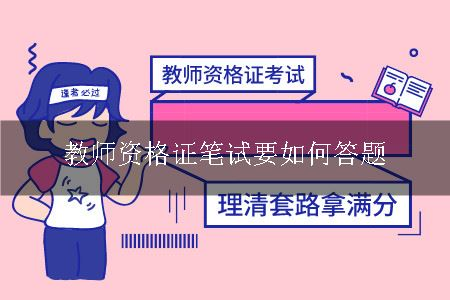# 教师资格证笔试要如何答题1、选择题。主要有三种方法：排错法、排正法、排他法。

2、简答题。根据所问问题的题型，对症下药。一般情况下，离不开“是什么”“为什么”“怎么办”这三点，考生回答出这三点并结合题意或或者自己的观点记性回答，一般情况下都能拿到较为可观的分数。

 幼儿教师资格证 小学教师资格证 中学教师资格证 综合素质 选择题(29×2=58)20min 材料分析题(3×14=42)40min 写作题(1×50=50)60min 保教知识与能力 选择题(10×3=30)10min 简答题(2×15=30)20min 论述题(1×20=20)20min 材料分析题(2×20=40)40min 活动设计题(1×30=30)30min —— —— 教育教学知识与能力 —— 选择题(20×2=40)15min 简答题(3×10=30)25min 材料分析题(2×20=40)40min 教学设计题(1×40=40)40min —— 教育知识与能力 —— —— 选择题(21×2=42)15min 辨析题(4×8=32)35min 简答题(4×10=40)30min 材料分析题(2×18=36)40min 学科知识与能力 —— —— 考生根据所考科目安排

## 高中教师资格证

•